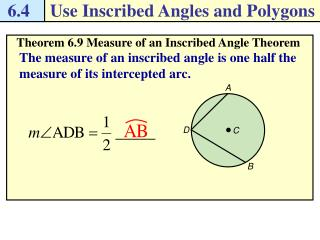DownloadDownload Presentation6.4

# 6.4

Télécharger la présentation## 6.4

- - - - - - - - - - - - - - - - - - - - - - - - - - - E N D - - - - - - - - - - - - - - - - - - - - - - - - - - -
##### Presentation Transcript

1. 6.4 Use Inscribed Angles and Polygons A D C B Theorem 6.9 Measure of an Inscribed Angle Theorem The measure of an inscribed angle is one half the measure of its intercepted arc.

2. 6.4 Use Inscribed Angles and Polygons Find the indicated measure in P. Q R P T S Use inscribed angles Example 1 Solution Because RQS is a semicircle,

3. 6.4 Use Inscribed Angles and Polygons H B F C G J D Checkpoint. Find the indicated measure.

4. 6.4 Use Inscribed Angles and Polygons A D B C Theorem 6.10 If two inscribed angles of a circle intercept the same arc, then the angles are congruent.

5. 6.4 Use Inscribed Angles and Polygons A D B C Theorem 6.11 If a right triangle is inscribed in a circle, then the hypotenuse is a diameter of the circle. Conversely, if one side of an inscribed triangle is a diameter of the circle, then the triangle is a right triangle and the angle opposite the diameter is the right angle. if and only if ____ is a diameter of the circle.

6. 6.4 Use Inscribed Angles and Polygons E F C G D D, E, F, and G lie in C if and only if Theorem 6.12 A quadrilateral can be inscribed in a circle if and only if its opposite angles are supplementary.

7. 6.4 Use Inscribed Angles and Polygons Use the circumscribed circle Example 2 Security A security camera that rotates 90o is placed in a location where the only thing viewed when rotating is the wall. You want to change the camera’s location. Where else can it be placed so that the wall is viewed in the same way? Solution From Theorem 6.11, you know that if a right triangle is inscribed in a circle, then the hypotenuse of the triangle is a ________ of the circle. diameter So, draw the circle that has the width of the wall as a _________. The wall fits perfectly with your camera’s 90 rotation from any point on the _________ in front of the wall. diameter semicircle

8. 6.4 Use Inscribed Angles and Polygons R Q S T Checkpoint. Complete the following exercises. By Theorem 6.10, if two inscribed angles of a circle intercept the same arc, then the angles are congruent.

9. 6.4 Use Inscribed Angles and Polygons Checkpoint. Complete the following exercises. • A right triangle is inscribed in a circle. The radius of the circle is 5.6 centimeters. What is the length of the hypotenuse of the right triangle? By Theorem 6.11, if a right triangle is inscribed in a circle, then the hypotenuse is a diameter of the circle.

10. 6.4 Use Inscribed Angles and Polygons Q R P S Use Theorem 6.12 Example 3 Find the value of each variable. Solution • PQRS is inscribed in a circle, so its opposite angles are _____________. supplementary

11. 6.4 Use Inscribed Angles and Polygons K L J M Use Theorem 6.12 Example 3 Find the value of each variable. Solution • JKLM is inscribed in a circle, so its opposite angles are _____________. supplementary

12. 6.4 Use Inscribed Angles and Polygons B A C D Checkpoint. Complete the following exercises.

13. 6.4 Use Inscribed Angles and Polygons Pg. 218, 6.4 #1-32